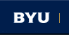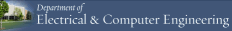BRIGHAM YOUNG UNIVERSITY
 Search BYUHome   |   Contact[expand all...]
[COLLAPSE ALL...]Matlab Tutorial for Beginners

# Introduction to Matlab. How to begin Matlab.

1. MATLAB is a numerical computing environment and programming language. Created by The MathWorks, MATLAB allows easy matrix manipulation, plotting of functions and data, implementation of algorithms, creation of user interfaces, and interfacing with programs in other languages. Many classes in our ECEn Department requires professional skill using matlab, such as ECEn 360, ECEn 370, ECEn 380, ECEn 483...This tutorial is for those who never touch matlab before or need some review for matlab.

2. This flash will teach you how to start matlab in BYU ECEn Department.
3. The topics below are the most important topics selected from the result of students' survey.

# Vectors, matrix and arrays

1. Setting up a vector or array
2. Useful default arrays
3. Operations between arrays
4. P.S. Matrix operations are very similar to the array operations, detailed information can be found in the attached document.

# Graphing

1. After setting and operating on arrays, the best way to see the behavior of x and y, or x,y, and z is to graph them. The most common functions for graphing are; plot, stem, polar, subplot, mesh, meshc, surf, surfc , and hold on.
2. plot and stem:
3. polar graph:
4. 3D graph:

# Derivative and Integration

1. Matlab can simulate both Integration and Derivative, not formulaically but by numerical approximation.
2. So, if you want a mathematical formula of a derivative, use a calculator or another program.
3. Derivative
4. Integral

# Signal processing

1. The number one important operation in simulating a system or signal is a convolution.
2. Before we look at a convolution, let's first simulate two very useful functions: the delta function and step function.
3. We can use long arrays storing zeros and ones to approximately simulate those two functions.
4. For example:
5. Now, we can look at the convolution. The syntax is just like this: conv(f1,f2);
6. For example
7. Filter and sampling processing will be taught in detail in ECEn380 class, you can also check it in the attached document.

# Help and look for function

1. The Help function is the most useful function in Matlab. It lists all primary help topics in the Command Window. Each main help topic corresponds to a directory name on the MATLAB search path.
2. The Syntax of help function is:
3. help /
4.         This command lists all operators and special characters, along with their descriptions.
5. help functionname
6.         This command displays M-file help, which is a brief description and the syntax for functionname, in the Command Window. The output includes a link to doc functionname, which displays the reference page in the Help browser, often providing additional information. I think that doc link is the most useful link in Matlab. It should contains all you want to know.

# A very convenient tool of MATLab 7.0 for graphing

## If you have any other questions, you can find the answers in this detailed matlab tutorial pdf file from BYU Department of Physics and Astronomy.

This tutorial is Written by: Zixu Zhu.        Email: sz_jamesrei@hotmail.comMaintained by ECEn IMMERSE Web Team.